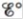# Calculate for the following half-reaction: AgI ( s ) + e − → Ag ( s ) + I − ( a q ) ( Hint: Reference the K sp value for AgI and the standard reduction potential for Ag + .)### Chemistry

10th Edition
Steven S. Zumdahl + 2 others
Publisher: Cengage Learning
ISBN: 9781305957404

#### Solutions

Chapter
Section### Chemistry

10th Edition
Steven S. Zumdahl + 2 others
Publisher: Cengage Learning
ISBN: 9781305957404
Chapter 18, Problem 89E
Textbook Problem
70 views

## Calculatefor the following half-reaction: AgI ( s ) + e − → Ag ( s ) + I − ( a q ) (Hint: Reference the Ksp value for AgI and the standard reduction potential for Ag+.)

Interpretation Introduction

Interpretation:

A half-cell reaction is given. The value of E° for the given cell reaction is to be calculated.

Concept introduction:

The relationship between reduction potential and standard reduction potential value and activities of species present in an electrochemical cell at a given temperature is given by the Nernst equation.

The value of Ecell is calculated using Nernst formula,

E=E°(RTnF)ln(Q)

At room temperature the above equation is specifies as,

E=E°(0.0591n)log(Q)

This relation is further used to determine the relation between ΔG° and K , ΔG° and E°cell .

Solubility product is applied only for those ionic compounds that are sparingly soluble. The product of solubility of ions is called solubility product and solubility is present in moles per liter.

To determine: The value of cell potential E° for the given cell reaction.

### Explanation of Solution

Given

The cell reaction is,

AgI(s)+eAg(s)+I(aq)

The value of standard reduction potential is,

Ag++eAgE°=0.80V

The value of solubility product constant is,

AgI(s)Ag+(aq)+I(aq)Ksp=8.3×1017

The reaction involves the transfer of 1 mole of electrons.

The standard cell potential at room temperature can be calculated by using the formula,

E°=0.0591nlogKsp

Where,

• Eo is the standard cell potential.
• n is the number of moles of electron transfer.
• Ksp is the solubility product constant.

Substitute the values of n and Ksp in the above equation,

E°=0.0591nlogKsp=0.05911log(8

### Still sussing out bartleby?

Check out a sample textbook solution.

See a sample solution

#### The Solution to Your Study Problems

Bartleby provides explanations to thousands of textbook problems written by our experts, many with advanced degrees!

Get Started